+
Standard Scores and Z-Scores
Central Tendency, Variation, and Distributions
0
of 90 possible points
Common Core: S.ID.4

# Standard Scores and Z-Scores

Author: Sophia Tutorial
##### Description:

Calculate a z-score using a given value and the corresponding mean and standard deviation.

(more)

Sophia’s self-paced online courses are a great way to save time and money as you earn credits eligible for transfer to many different colleges and universities.*

No credit card required

46 Sophia partners guarantee credit transfer.

299 Institutions have accepted or given pre-approval for credit transfer.

* The American Council on Education's College Credit Recommendation Service (ACE Credit®) has evaluated and recommended college credit for 33 of Sophia’s online courses. Many different colleges and universities consider ACE CREDIT recommendations in determining the applicability to their course and degree programs.

Tutorial
what's covered
This tutorial will cover the topic of z-scores. Our discussion breaks down as follows:

1. Z-Scores
1. Calculating Z-Scores
2. Negative Z-Scores
2. Converting Standard Distributions to Z-Scores

## 1. Z-Scores

Z-scores are values that allow you to make one-to-one comparisons between a couple of different distributions. Often, you want to compare two things, but it's not really fair to compare them directly. Z-scores help with this.

A z-score is a value that explains how many standard deviations away from the mean an observation is. It can be positive (if the value is above the mean) or negative (if the value is below the mean).

### IN CONTEXT

Suppose you are taking a math class. For the first exam of the year, the class mean was 88 points, and the standard deviation was 5. You scored a 92, so you did better than the class average. The second test was much harder. You scored an 80, which is a lot worse than you did the first time, but the class mean was 74. So, even though your score went down, the class average also went down. The standard deviation, this time, was 4 points. Did you do better on the first or the second test, relative to your classmates?

It's obvious that you scored higher on the first test, but relative to your classmates, did you do better on the first test or the second test? Just based on the scores, it's not fair to say that you did better on the first test. You want to see how you did relative to your classmates. Z-scores are going to allow you to make this comparison.

Z-scores are sometimes called standardized scores because they measure how many standard deviations away from the mean your observation is. In the previous example, for the first test of the year, the standard deviation was 5 for the first exam. You scored 4 points higher than average, 92 compared to the average score of 88. This means that your z-score is less than 1 because you are less than one standard deviation above the mean.

term to know
Standard Scores/Z-Scores
A value that explains how many standard deviations away from the mean an observation is. It can be positive (if the value is above the mean) or negative (if the value is below the mean).
1a. Calculating Z-Scores

In the above example, the actual z-score for the first test is positive 4/5: positive 4 means above the mean, and divided by 5, the standard deviation.

But how do you get this calculation? You use this formula:

formula
Z-ScoreThe z-score (represented by z) is equal to the raw score (x) minus the mean (mu), divided by the standard deviation (sigma).

try it
Using our example above, let's compare the standardized scores by comparing the z-score from the first test to the z-score from the second. First, let's calculate the z-score from the first test, using our formula:

Comparing Z-Scores of Two Tests
Test 1 The class mean is 88 with a standard deviation of 5.

You score a 92.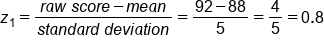Test 2 The class mean is 74 with a standard deviation of 4.

You score an 80.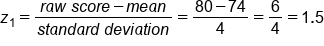What does this mean? Because positive 1.5 is larger than positive 0.8, your score on the second test was, in fact, better relative to the rest of the class.

1b. Negative Z-Scores
The other thing that's worth noting is that a z-score can be negative. If you're subtracting a bigger number from a smaller number (i.e., if the raw score is a smaller number than the mean), then you'll end up with a negative value.

big idea
If the raw score is below the mean, the z-score will be negative. If the raw score is above the mean, the z-score is positive. If the raw score and the mean are the same, the z-score is 0.

## 2. Converting Standard Distributions to Z-Scores

How are z-scores used in standard distributions? Let's explore an example.

EXAMPLE

Men's heights follow a normal distribution, with a mean of 68 and a standard deviation of 3.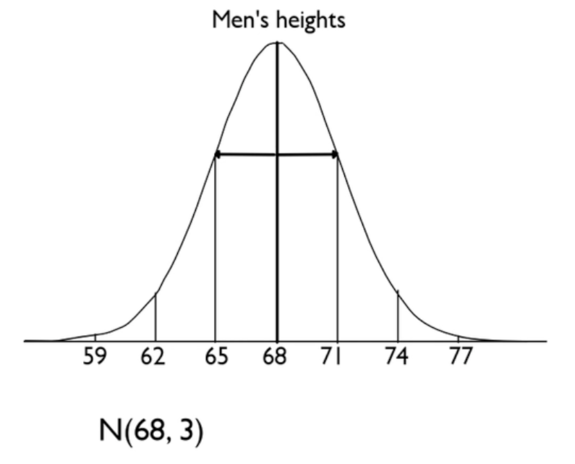Imagine, then, converting the 59, 62, 65, etc., into z-scores. For example: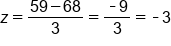A z-score of negative 3 tells us that 59 is three standard deviations below the mean. If we convert all these values into z-scores, what would that normal distribution look like?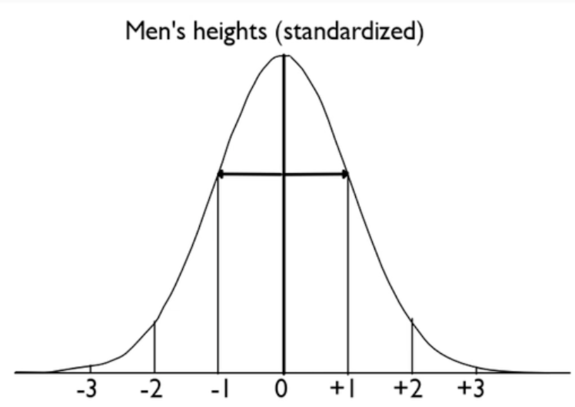Since the noted numbers are integers of standard deviations away from the mean, and that's what z-scores measure, each can be represented by z-scores.

big idea
This normal distribution of z-scores is called the standard normal distribution--standard, because it's the normal distribution of standardized scores.

summary
Standardized scores / z-scores allow you to make one-to-one comparisons of scores from one distribution to scores from another distribution. They measure how many standard deviations above or below the mean you are, and thus normal distributions can be converted to z-scores. A point that's further above the mean will have a higher z-score than a point that's closer to the mean. A point above the mean will have a positive z-score, and a point below the mean will have a negative z-score.

Good luck!

Source: Adapted from Sophia tutorial by Jonathan Osters.

Terms to Know
Standard Scores/z-scores

A value that explains how many standard deviations away from the mean an observation is. It can be positive (if the value is above the mean) or negative (if the value is below the mean).

Formulas to Know
z-scoreRating